## Tuesday, 26 June 2012

### Really Fast I/O methods for Programming in C++ (Input Methods)..

In programming minimizing the runtime of your program is very necessary as the time limit of the problems is very strict..

Many times I have come across the warning – “Be Careful .. Large Input Output”…
In such problems the optimization in the input/output method really helps in giving a better runtime…
After surfing a lot on the web and reading about the different methods, I made a template for myself, which I use in all my problems and sometimes it help me in getting on the 1st page of best solutions ;)

You can check all the Fast I/O methods on - www.spoj.pl/problems/INTEST (Input test)
www.spoj.pl/problems/INOUTEST (Input + Output Test)

Due to simplicity most of C++ programmers use cin/cout for the Input/Output...
But when the testcases are large cin/cout doesn't work.. You can try it on the above problems...
A solution with cin/cout will time out...

So one of the alternate is the scanf/printf which is nearly 3 times faster than the cin/cout...
An Accepted solution of the problem INTEST is

__________________________________________________________________________________

#include<stdio.h>
int main()
{
int n,k;
scanf("%d%d",&n,&k);
int cnt=0;
while(n--)
{
int num;
scanf("%d",&num);
if(num%k==0)cnt++;
}
printf("%d",cnt);
return 0;
}

__________________________________________________________________________________
But its runtime is 5.52 seconds which is not very good...
So we search for other alternates...

Another faster input handling method is the getchar_unlocked()...
getchar_unlocked is thread unsafe as it locks the screen while getting the input.. But using it on the spoj server will not harm your screen :D....
It is easy to implemet and is very fast...
We can use getchar_unlocked to handle integers and strings faster...

An Implementation of getting integer input through getchar_unlocked() -
Accepted INTEST Solution
_______________________________________________________________________________

#include<iostream>
#include<cstdio>
#define gc getchar_unlocked

void scanint(int &x)
{
register int c = gc();
x = 0;
for(;(c<48 || c>57);c = gc());
for(;c>47 && c<58;c = gc()) {x = (x<<1) + (x<<3) + c - 48;}
}

int main()
{

int n,k;
scanint(n);
scanint(k);
int cnt=0;
while(n--)
{
int num;
scanint(num);
if(num%k==0)cnt++;
}
printf("%d",cnt);
return 0;
}

__________________________________________________________________________________

And the runtime of this solution is  0.90 secs.. which is much faster than the scanf...

Registers are faster than memory to access, so the variables which are most frequently used in a C program can be put in registers using register keyword...

This implementation was for positive integers.. Similarly you can make one for the +/- integers..

___________________________________________________________________________________

void scanint(int &x)
{
register int c = gc();
x = 0;
int neg = 0;
for(;((c<48 || c>57) && c != '-');c = gc());
if(c=='-') {neg=1;c=gc();}
for(;c>47 && c<58;c = gc()) {x = (x<<1) + (x<<3) + c - 48;}
if(neg) x=-x;
}

___________________________________________________________________________________

So this is a very easy to implement fast Input handling method....

We have another fast Input method that is - fread or fread_unlocked.......
There isn't much difference between fread and fread_unlocked as in both the whole input file is first taken in an input buffer and then processed.. Main difference is the locking of screen in fread_unlocked...

Implementaion of fread in INTEST ...
__________________________________________________________________________________

#include<cstdio>
#include<iostream>
#include<cstdlib>
using namespace std;
#define size 65536
int main()
{

char b[size];
int t=0,n,k,cnt=0;
int c,j;
scanf("%d %d\n",&n,&k);
while((c = fread(b,1,size,stdin))>0)
{
for(j=0;j<c;j++)
{
if(b[j]=='\n')
{
if(t%k==0)cnt++;
t = 0;
}
else
{
t = (t*10) + (b[j]-'0');
}
}
}
printf("%d\n",cnt);
return 0;
}

__________________________________________________________________________________

Now this method is more faster as the runtime of this one is 0.65 secs...
Using fread_unlocked increased the runtime to 0.78 secs...
So better use fread()....

But I found getchar_unlocked() much easier to manipulate and code.. So that's my choice...
These were few Input methods that I found useful.. For string handling gets() and fgets() are also good....

If there are other Input handling methods, please let me know....

I will discuss the output methods with the implementations in my next Blog....... :):)

Till then..
Love and Live..
As Life is Small.. :) :)

#### 32 comments:

1.Awesome... Thanks Abhi... :)

2.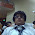3.for your fread example, i think you won't read the entire input, fread will return a zero if less than "size" bytes are read, thus interrupting your loop in the middle, isn't that right?

4.getchar_unlocked is undeclared function on my system...i'm usin "dev c++" and also "code blocks" both showing same error..please help me!!

1.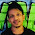compile directly on ideone

2.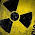use getchar() .. getchar_unlocked() is available on linux not on windows.

5.how to make the change in this function to get faster i/p for char ?

6.Fast i/o for char??

7.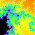Please see this stackoverflow thread: http://stackoverflow.com/questions/20802428/fast-input-output-in-c Thanks.

8.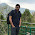Good analysis on faster I/O in c/c++

9.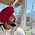that was really helpful.thanks

10.I didn't understand this :

for(;(c<48 || c>57);c = gc());
for(;c>47 && c<58;c = gc()) {x = (x<<1) + (x<<3) + c - 48;}

Can someone explain what that does?

1.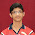I will try to explain this.

The First line is simply to reject every character entry which is not a digit.

The second line is simply a magical line with a lot of importance.

For Example: The Input is: 12. Then normally, if we are taking it character by character, first 1 will be encountered. After that, 2 will be encountered. So, logically, to represent the number in integer form, After taking 1 as input, we have to multiply 1 by 10 and then add the next character to the variable to make it 12, i.e. (10+2).

The same thing is done here.

The alternative way is "for(;c>47 && c<58;c = gc()) {x = x*10 + c - 48;}"

But the logic given by the author is faster because shifting operator is much faster than the multiplication operator.

Here is the explanation of what the author has done:
x=(x<<1)+(x<<3)+(c-48);

Explanation for expression in between Parentheses 1 & 2:
As you know, left shifting by every bit results multiplication by 2. So, shifting by 1 bit has same effect as that of x*2 and shifting by 3 bit has same effect as x*2*2*2=x*8.

Explanation for expression in between Parentheses 3:
Simply converting character into integer digit.

2.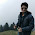Awesome man

3.11.how to run the fread example in ubuntu?? where you are decreasing the value of n??

12.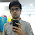how to use getchar_unlocked for strings?
could you provide the code or some link?
i need it urgently....

1.inline void fastRead_string(char *str)
24
{
25
register char c = 0;
26
register int i = 0;
27

28
while (c < 33)
29
c = getchar_unlocked();
30

31
while (c != '\n') {
32
str[i] = c;
33
c = getchar_unlocked();
34
i = i + 1;
35
}
36

37
str[i] = '\0';
38
}

13.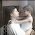u can use fread() to enter strings

#include
#include
#define gc getchar_unlocked

void scanint(int &x)
{
register int c = gc();
x = 0;
for(;(c<48 || c>57);c = gc()){x=c;}
for(;c>47 && c<58;c = gc()) {x = (x<<1) + (x<<3) + c - 48;}
}

int main()
{

int n,k;
scanint(n);
scanint(k);
int cnt=0;
while(n--)
{
int num;
scanint(num);
if(num%k==0)cnt++;
} char nu;
fread(&nu,1,1,stdin);
printf("%d %c ",cnt,nu);
return 0;
}

14.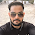why ur program give an error on dev c++ complier
error "gc was not declared in this scope"

1.This comment has been removed by the author.

2.This comment has been removed by the author.

3.getchar_unlocked is not a C or C++ standard function and therefore it doesn't work on Windows. It is a POSIX(Portable Operating System Interface) standard , but Windows compilers don't support all POSIX functions.
Ue getchar insted of getchar_unlocked .

15.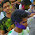#define gc getchar_unlocked

16.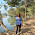thnkew so much :)

17.Good info on this post. You have shared very valuable info here.

c programming training

18.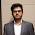Thanks Bro :)

19.really helpful.. Thanks

20.21.Its fantastic explaintion lot of information gather it...nice article....
seo company in Chennai

22.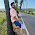explain more about fread

23.the above information is for c developers, please tell us more about c++ input methods.